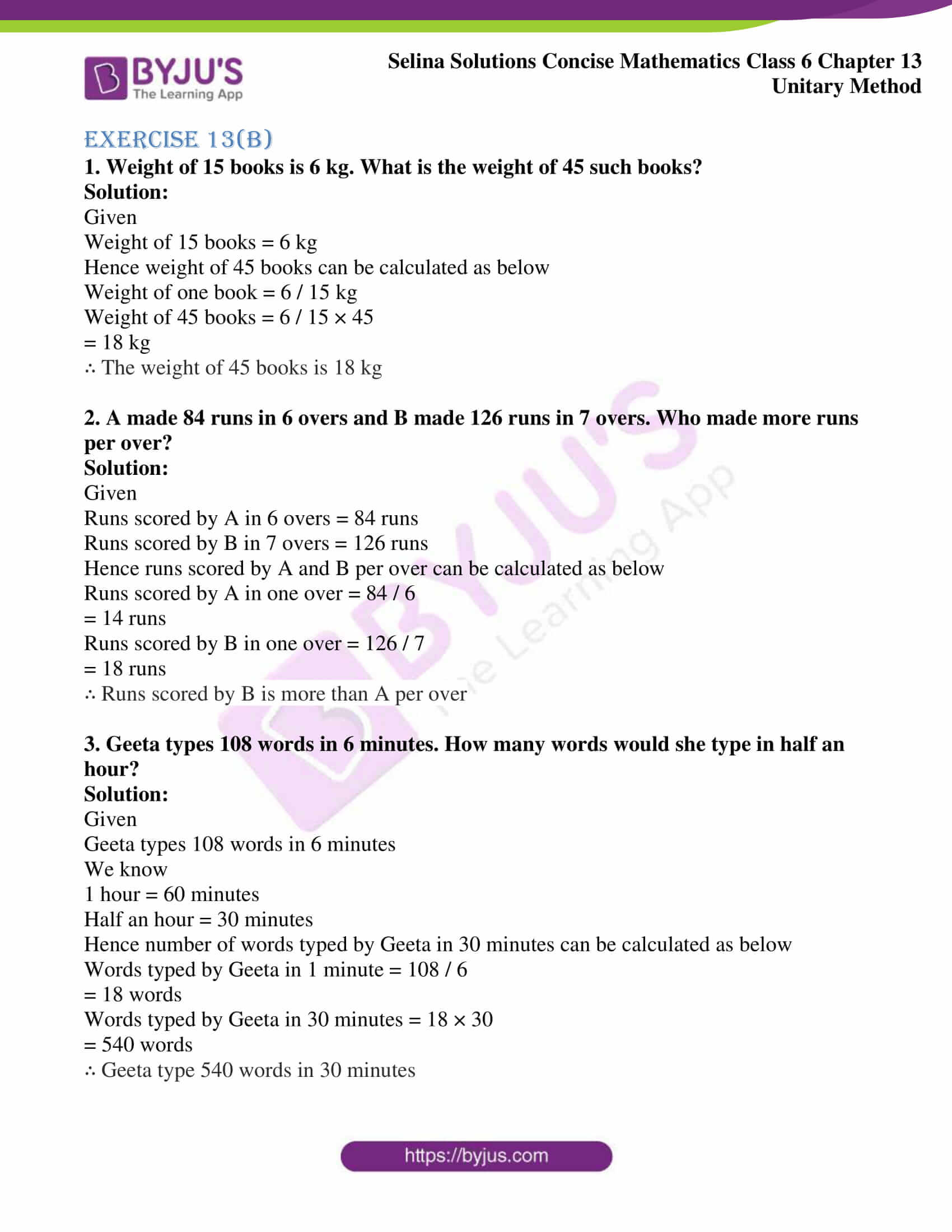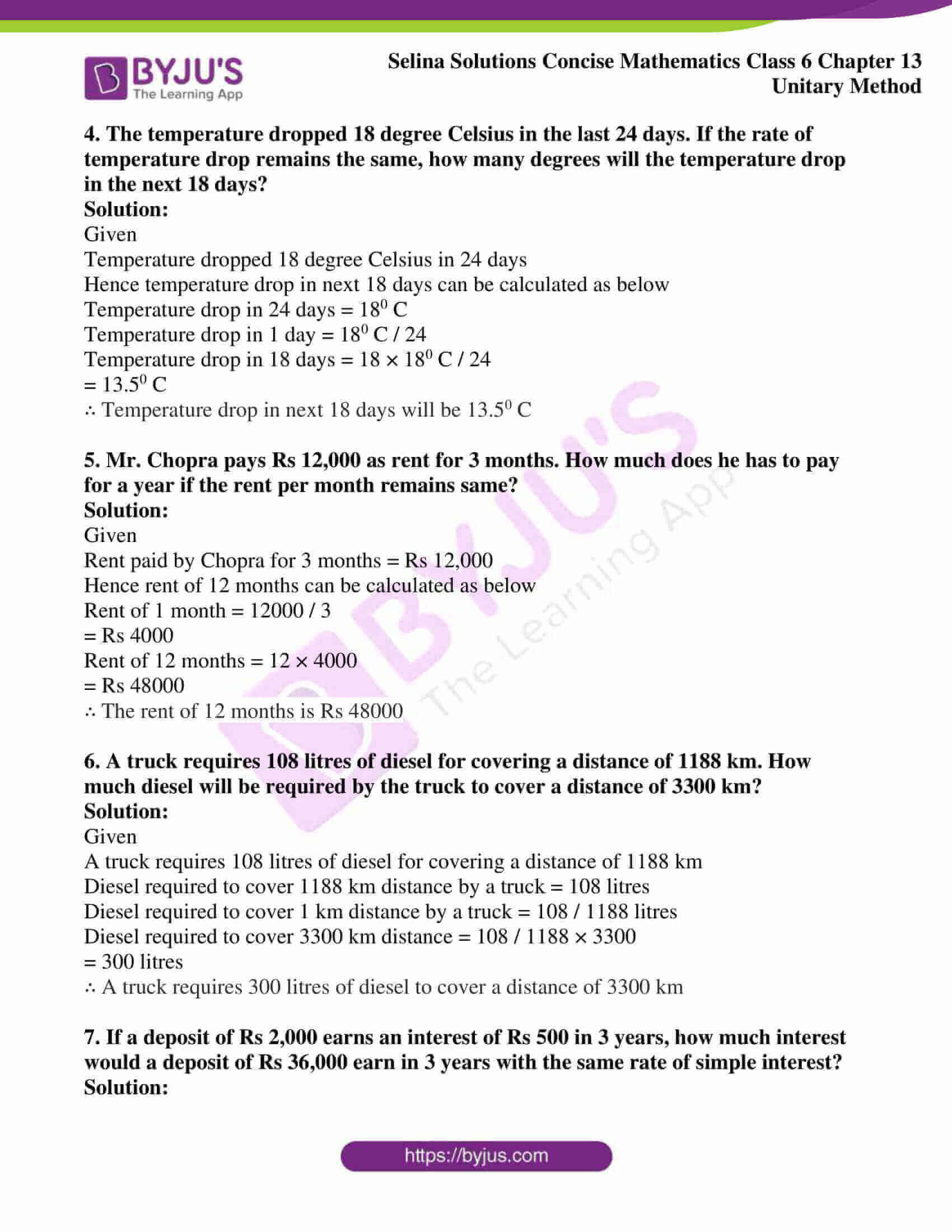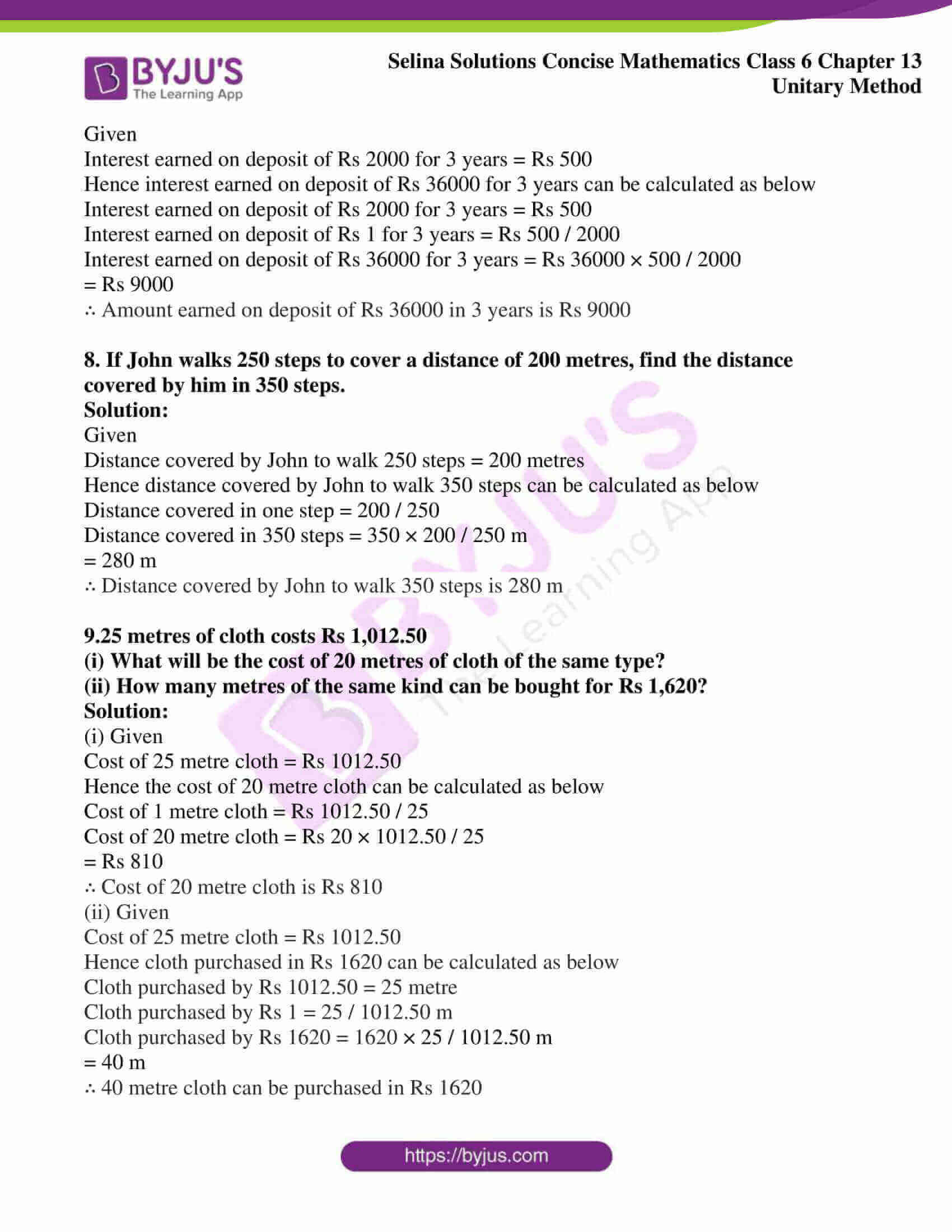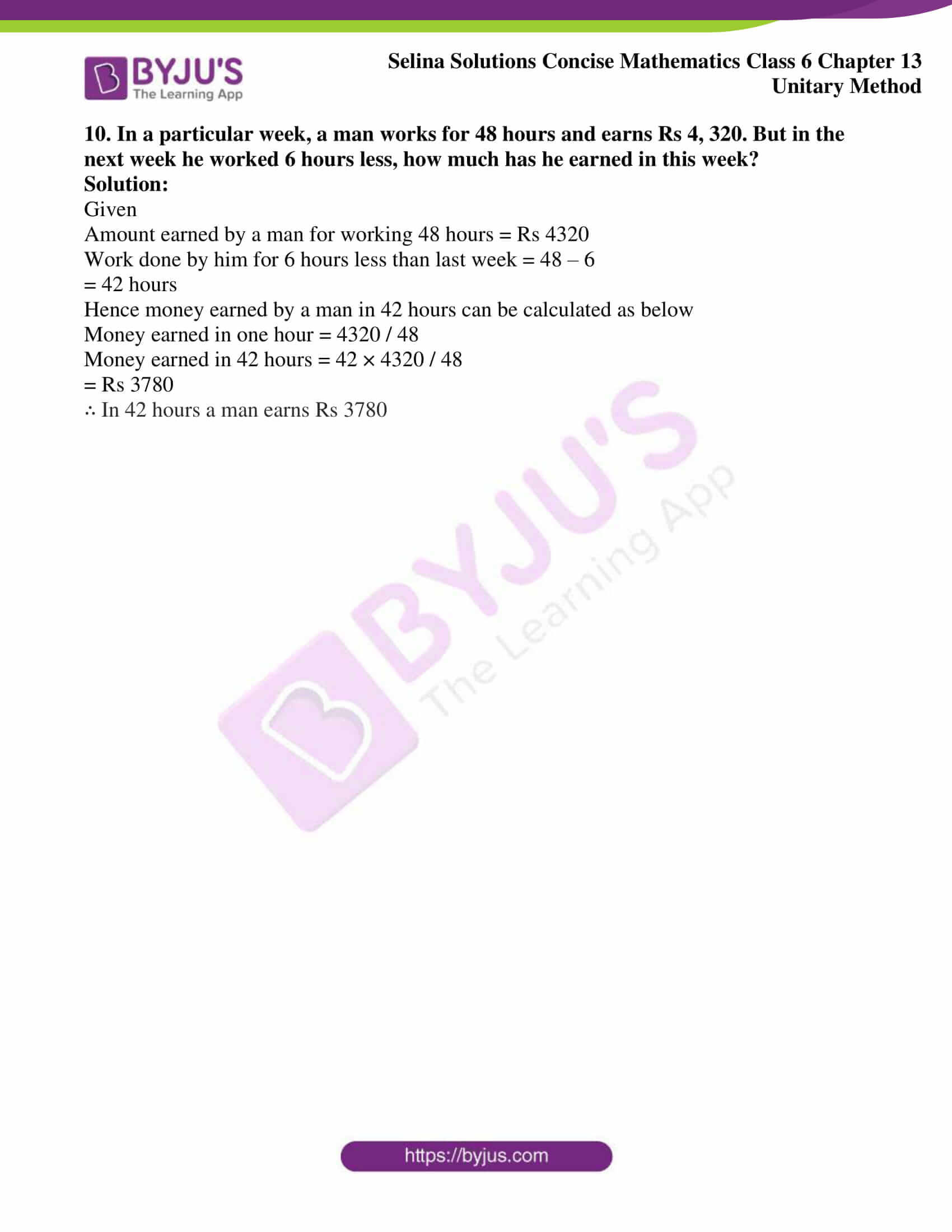# Selina Solutions Concise Mathematics Class 6 Chapter 13: Unitary Method Exercise 13(B)

Selina Solutions Concise Mathematics Class 6 Chapter 13: Unitary Method Exercise 13(B) provides students a better idea of solving the exercise wise problems quickly. The solutions are designed by the faculty at BYJU’S, with the intention to enhance interest among students in Mathematics. Solutions PDF are available for students 24/7, which help them in clearing their doubts on the spot. To know more about the concepts, students can use Selina Solutions Concise Mathematics Class 6 Chapter 13 Unitary Method Exercise 13(B) PDF, from the links which are provided below.

## Selina Solutions Concise Mathematics Class 6 Chapter 13: Unitary Method Exercise 13(B) Download PDF### Access another exercise of Selina Solutions Concise Mathematics Class 6 Chapter 13 Unitary Method

Exercise 13(A) Solutions

### Access Selina Solutions Concise Mathematics Class 6 Chapter 13: Unitary Method Exercise 13(B)

#### Exercise 13(B)

1. Weight of 15 books is 6 kg. What is the weight of 45 such books?

Solution:

Given

Weight of 15 books = 6 kg

Hence weight of 45 books can be calculated as below

Weight of one book = 6 / 15 kg

Weight of 45 books = 6 / 15 × 45

= 18 kg

∴ The weight of 45 books is 18 kg

2. A made 84 runs in 6 overs and B made 126 runs in 7 overs. Who made more runs per over?

Solution:

Given

Runs scored by A in 6 overs = 84 runs

Runs scored by B in 7 overs = 126 runs

Hence runs scored by A and B per over can be calculated as below

Runs scored by A in one over = 84 / 6

= 14 runs

Runs scored by B in one over = 126 / 7

= 18 runs

∴ Runs scored by B is more than A per over

3. Geeta types 108 words in 6 minutes. How many words would she type in half an hour?

Solution:

Given

Geeta types 108 words in 6 minutes

We know

1 hour = 60 minutes

Half an hour = 30 minutes

Hence number of words typed by Geeta in 30 minutes can be calculated as below

Words typed by Geeta in 1 minute = 108 / 6

= 18 words

Words typed by Geeta in 30 minutes = 18 × 30

= 540 words

∴ Geeta type 540 words in 30 minutes

4. The temperature dropped 18 degree Celsius in the last 24 days. If the rate of temperature drop remains the same, how many degrees will the temperature drop in the next 18 days?

Solution:

Given

Temperature dropped 18 degree Celsius in 24 days

Hence temperature drop in next 18 days can be calculated as below

Temperature drop in 24 days = 180 C

Temperature drop in 1 day = 180 C / 24

Temperature drop in 18 days = 18 × 180 C / 24

= 13.50 C

∴ Temperature drop in next 18 days will be 13.50 C

5. Mr. Chopra pays Rs 12,000 as rent for 3 months. How much does he has to pay for a year if the rent per month remains same?

Solution:

Given

Rent paid by Chopra for 3 months = Rs 12,000

Hence rent of 12 months can be calculated as below

Rent of 1 month = 12000 / 3

= Rs 4000

Rent of 12 months = 12 × 4000

= Rs 48000

∴ The rent of 12 months is Rs 48000

6. A truck requires 108 litres of diesel for covering a distance of 1188 km. How much diesel will be required by the truck to cover a distance of 3300 km?

Solution:

Given

A truck requires 108 litres of diesel for covering a distance of 1188 km

Diesel required to cover 1188 km distance by a truck = 108 litres

Diesel required to cover 1 km distance by a truck = 108 / 1188 litres

Diesel required to cover 3300 km distance = 108 / 1188 × 3300

= 300 litres

∴ A truck requires 300 litres of diesel to cover a distance of 3300 km

7. If a deposit of Rs 2,000 earns an interest of Rs 500 in 3 years, how much interest would a deposit of Rs 36,000 earn in 3 years with the same rate of simple interest?

Solution:

Given

Interest earned on deposit of Rs 2000 for 3 years = Rs 500

Hence interest earned on deposit of Rs 36000 for 3 years can be calculated as below

Interest earned on deposit of Rs 2000 for 3 years = Rs 500

Interest earned on deposit of Rs 1 for 3 years = Rs 500 / 2000

Interest earned on deposit of Rs 36000 for 3 years = Rs 36000 × 500 / 2000

= Rs 9000

∴ Amount earned on deposit of Rs 36000 in 3 years is Rs 9000

8. If John walks 250 steps to cover a distance of 200 metres, find the distance covered by him in 350 steps.

Solution:

Given

Distance covered by John to walk 250 steps = 200 metres

Hence distance covered by John to walk 350 steps can be calculated as below

Distance covered in one step = 200 / 250

Distance covered in 350 steps = 350 × 200 / 250 m

= 280 m

∴ Distance covered by John to walk 350 steps is 280 m

9.25 metres of cloth costs Rs 1,012.50

(i) What will be the cost of 20 metres of cloth of the same type?

(ii) How many metres of the same kind can be bought for Rs 1,620?

Solution:

(i) Given

Cost of 25 metre cloth = Rs 1012.50

Hence the cost of 20 metre cloth can be calculated as below

Cost of 1 metre cloth = Rs 1012.50 / 25

Cost of 20 metre cloth = Rs 20 × 1012.50 / 25

= Rs 810

∴ Cost of 20 metre cloth is Rs 810

(ii) Given

Cost of 25 metre cloth = Rs 1012.50

Hence cloth purchased in Rs 1620 can be calculated as below

Cloth purchased by Rs 1012.50 = 25 metre

Cloth purchased by Rs 1 = 25 / 1012.50 m

Cloth purchased by Rs 1620 = 1620 × 25 / 1012.50 m

= 40 m

∴ 40 metre cloth can be purchased in Rs 1620

10. In a particular week, a man works for 48 hours and earns Rs 4, 320. But in the next week he worked 6 hours less, how much has he earned in this week?

Solution:

Given

Amount earned by a man for working 48 hours = Rs 4320

Work done by him for 6 hours less than last week = 48 – 6

= 42 hours

Hence money earned by a man in 42 hours can be calculated as below

Money earned in one hour = 4320 / 48

Money earned in 42 hours = 42 × 4320 / 48

= Rs 3780

∴ In 42 hours a man earns Rs 3780